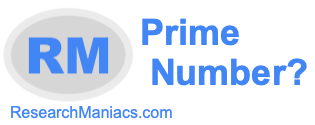Is 1 a prime number?To find out if 1 is a prime number, we went through our prime number check list. Everything on our prime number check list must be true for 1 to be a prime number.

For 1 to be a prime number, it must be an integer and a positive number: True

For 1 to be a prime number, it must be a number greater than 1: False

For 1 to be a prime number, it must be the number 2 or an odd number greater than 2: False

For 1 to be a prime number, it must only be divisible by 1 and itself: False

All of our tests above did not prove true, therefore the answer to the question: "Is 1 a prime number?" is as follows:

NO: 1 is not a prime number.

What is a prime number?
Enter another number below to see if it is a prime number.

Is 2 a prime number?
Using the knowledge you learned above to figure out if 1 is a prime number, do you know the answer the next number on our list?